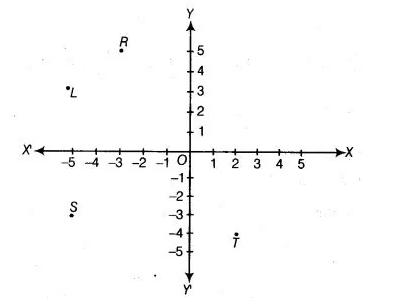# In following figure, the point identified by the coordinates (-5, 3) is`
Question:

In following figure, the point identified by the coordinates (-5, 3) is(a) $T$

(b) $R$

(c) $L$

(d) $S$

Solution:

(c) In point (-5, 3), x-coordinate is negative and y-coordinate is positive, so it will lie in II quadrant. Now, we see that perpendicular distance of L from V-axis is 5 and from X-axis is 3. So, the required point is L.